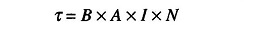## Permanent Magnet Moving Coil Instrument (PMMC):

In this type of Permanent Magnet Moving Coil Instrument (PMMC), we have a coil suspended in the magnetic field of a permanent magnet in the shape of a horse-shoe. The coil is suspended so that it can rotate freely in the magnetic field. When current flows in the coil, the developed (electromagnetic) torque causes the coil to rotate.

The electromagnetic (EM) torque is counterbalanced by a mechanical torque of control springs attached to the movable coil. The balance of torques, and therefore the angular position of the movable coil is indicated by a pointer against a fixed reference called a scale. The equation for the developed torque of PMMC, derived from the basic law for electromagnetic torque iswhere

• τ = torque, Newton-meter
• B = flux density in the air gap, Wb/m2
• A = effective coil area (m2)
• N = number of turns of wire of the coil
• I = current in the movable coil (amperes)

The PMMC equation shows that the developed torque is proportional to the flux density of the field in which the coil rotates, the current coil constants (area and number of turns). Since both flux density and coil constants are fixed for a given instrument, the developed torque is a direct indication of the current in the coil. The pointer deflection can therefore be used to measure current.

Scroll to Top